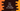# Find the area of a triangle in C++## Area of a triangle in C++ :

In this C++ program, we will learn how to find the area of a triangle if its sides are given. We will take the sides as input from the user. This example will show you how to read user inputs in C++ and how to do mathematical calculations.

Before moving to the program, let me quickly show you the mathematical formula to calculate the triangle area.

### Formula to calculate the area of a triangle :

We need the sides of a triangle to calculate its area. For example, if the sides of a triangle are s1, s2 and s3, its area is square root of s * (s - s1) * (s - s2) * (s - s3), where s = (s1 + s2 + s3)/2.

We will get the sides as inputs from the user and then the program will calculate the final area. We will also add two validation checks in the program :

1. All sides should be positive.
2. The sum of any two sides should be always greater than the third side.

These are basic validation check for a valid triangle. We will write different functions to do these validations.

Let’s code :

### C++ program to find the area of a triangle :

``````#include<iostream>
#include<cmath>
using namespace std;

// 1
void isSideValid(float length){
if(length< 0){
cout<<"Error !! Please enter a valid side"<<endl;;
exit(0);
}
}

// 2
void checkAllSides(float first, float second, float third){
if(first + second <= third || first + third <= second || second + third <= first){
cout<<"Error !! Sides are not valid"<<endl;
exit(0);
}
}

int main()
{
// 3
float firstSide, secondSide, thirdSide;
float s,area;

// 4
cout<< "Enter the length of the first side : "<<endl; cin>>firstSide;
isSideValid(firstSide);

cout<< "Enter the length of the second side : "<<endl; cin>>secondSide;
isSideValid(secondSide);

cout<< "Enter the length of the third side : "<<endl; cin>>thirdSide;
isSideValid(thirdSide);

// 5
checkAllSides(firstSide,secondSide,thirdSide);

// 6
s = (firstSide + secondSide + thirdSide)/2;

// 7
area = sqrt(s * (s - firstSide) * (s - secondSide) * (s - thirdSide));

cout << "Area of the triangle is "<<area<<endl;
return 0;
}  ``````

### Explanation :

The commented numbers in the above program denote the step numbers below :

1. isSideValid function is used to check if a side entered by the user is valid or not. If it is less than 0, it exits the program.
2. checkAllSides function checks if the three sides entered by the user are valid or not. If not valid, it exits the program.
3. Create some floating point variables to store the sides of the triangle, s and final area of the triangle.
4. Ask the user to enter the length of each side. Read the values and also check if each one is valid or not.
5. Once all sides are read, use checkAllSides function to verify if the sides are valid.
6. Calculate the value of s.
7. Calculate the area and print this value.

### Sample Output :

``````Enter the length of the first side :
5
Enter the length of the second side :
4
Enter the length of the third side :
3
Area of the triangle is 6

Enter the length of the first side :
10
Enter the length of the second side :
12
Enter the length of the third side :
13
Area of the triangle is 56.9951``````